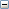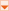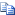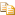﻿ MeasureVertHistogram MethodCollapse All Expand All
 CiTools Object : MeasureVertHistogram Method

#Description

Measure vertical histogram

#Syntax

Visual Basic
`Public Function MeasureVertHistogram() As Variant`

#Remarks

!MISSING PHRASE '_COLORFAIL'!

#Example

Measure Vertical and Horizontal Histograms
VB Example (Visual Basic)Copy Code
```Public Sub T_MeasureHistogram(ByRef Tools As CiTools)
Dim Hist, i, start

Hist = Tools.MeasureHorzHistogram
For i = LBound(Hist) To UBound(Hist)
start = 0:  If (Tools.Image.IsZone) Then start = Tools.Image.Zone.top
Debug.Print "Row=" & start + i & " Pixels=" & Hist(i)
Next

Hist = Tools.MeasureVertHistogram
For i = LBound(Hist) To UBound(Hist)
start = 0:  If (Tools.Image.IsZone) Then start = Tools.Image.Zone.left
Debug.Print "Col=" & start + i & " Pixels=" & Hist(i)
Next
End Sub```